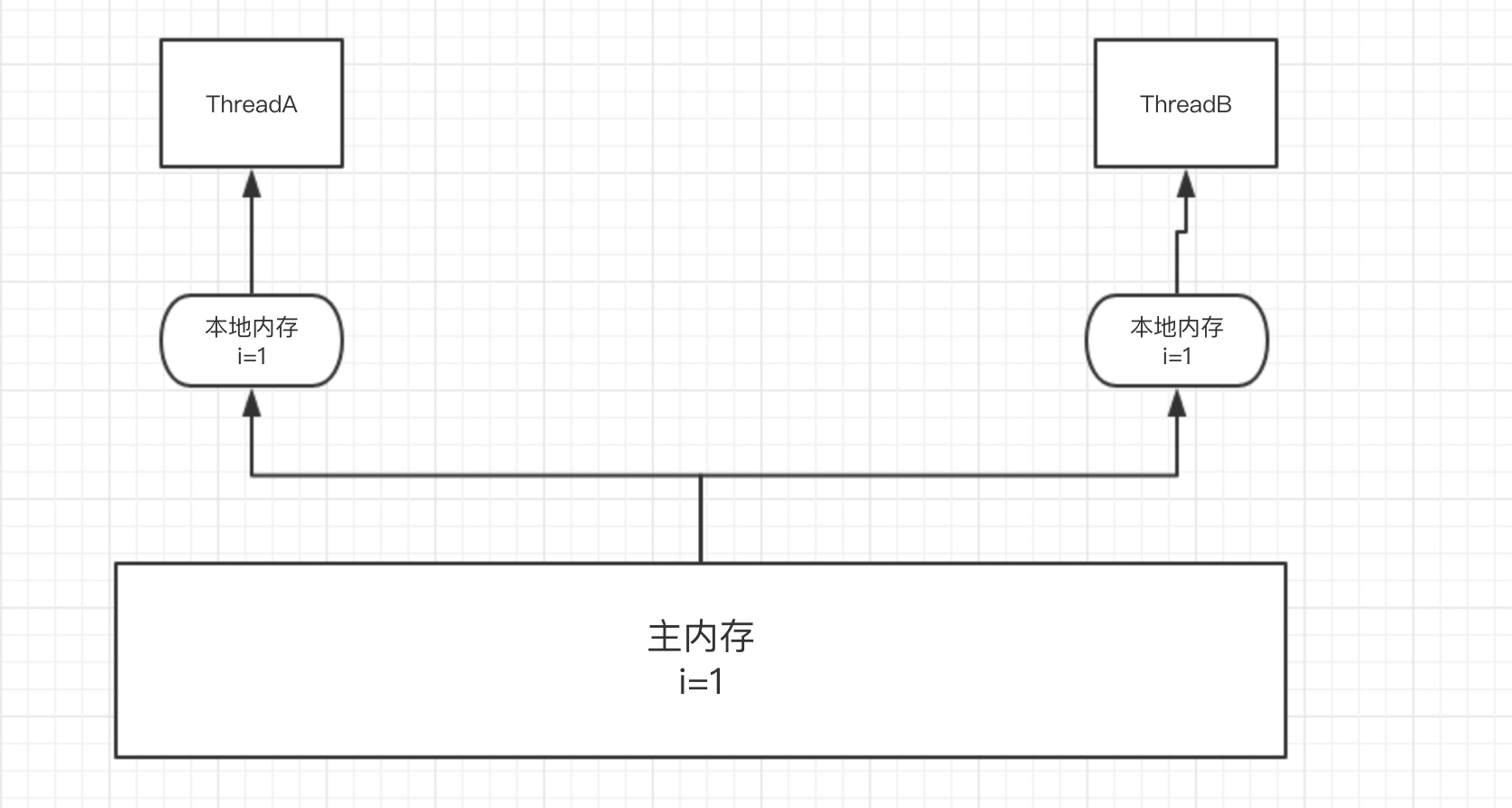# Java volatile关键字

## volatile

Posted by crossoverjie on December 29, 2020

## 内存可见性`volatile` 修饰之后并不是让线程直接从主内存中获取数据，依然需要将变量拷贝到工作内存中

### 内存可见性的应用

``````public class Volatile implements Runnable{

private static volatile boolean flag = true ;

@Override
public void run() {
while (flag){
}
}

public static void main(String[] args) throws InterruptedException {
Volatile aVolatile = new Volatile();

System.out.println("main 线程正在运行") ;

Scanner sc = new Scanner(System.in);
while(sc.hasNext()){
String value = sc.next();
if(value.equals("1")){

@Override
public void run() {
}
}).start();

break ;
}
}

System.out.println("主线程退出了！");

}

flag = false ;
}

}
``````

`volatile` 修饰的变量进行并发操作是线程安全的。

``````public class VolatileInc implements Runnable{

private static volatile int count = 0 ; //使用 volatile 修饰基本数据内存不能保证原子性

//private static AtomicInteger count = new AtomicInteger() ;

@Override
public void run() {
for (int i=0;i<10000 ;i++){
count ++ ;
//count.incrementAndGet() ;
}
}

public static void main(String[] args) throws InterruptedException {
VolatileInc volatileInc = new VolatileInc() ;
t1.start();
//t1.join();

t2.start();
//t2.join();
for (int i=0;i<10000 ;i++){
count ++ ;
//count.incrementAndGet();
}

System.out.println("最终Count="+count);
}
}
``````

• 所以想到达到线程安全可以使这三个线程串行执行(其实就是单线程，没有发挥多线程的优势)。

• 也可以使用 `synchronized` 或者是锁的方式来保证原子性。

• 还可以用 `Atomic` 包中 `AtomicInteger` 来替换 `int`，它利用了 `CAS` 算法来保证了原子性。

## 指令重排

``````int a=10 ;//1
int b=20 ;//2
int c= a+b ;//3
``````

``````private static Map<String,String> value ;
private static volatile boolean flag = fasle ;

//以下方法发生在线程 A 中 初始化 Map
public void initMap(){
//耗时操作
value = getMapValue() ;//1
flag = true ;//2
}

//发生在线程 B中 等到 Map 初始化成功进行其他操作
public void doSomeThing(){
while(!flag){
sleep() ;
}
//dosomething
doSomeThing(value);
}

``````

### 指令重排的的应用

``````public class Singleton {

private static volatile Singleton singleton;

private Singleton() {
}

public static Singleton getInstance() {
if (singleton == null) {
synchronized (Singleton.class) {
if (singleton == null) {
//防止指令重排
singleton = new Singleton();
}
}
}
return singleton;
}
}
``````

• 分配内存空间。(1)
• 初始化对象。(2)
• `singleton` 对象指向分配的内存地址。(3)

## 总结

`volatile``Java` 并发中用的很多，比如像 `Atomic` 包中的 `value`、以及 `AbstractQueuedLongSynchronizer` 中的 `state` 都是被定义为 `volatile` 来用于保证内存可见性。